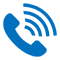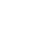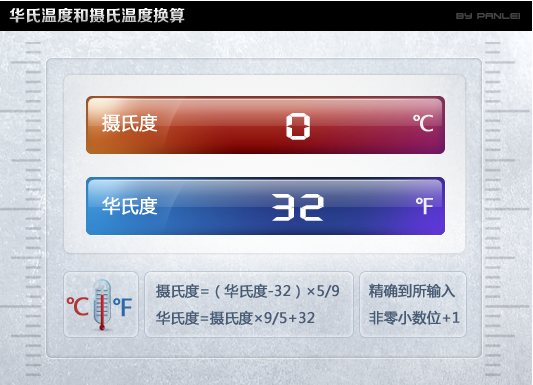24小时服务热线：

18627771137

### 空压机产品分类24小时服务热线：

18627771137

# 空压机常用单位换算表

2018-09-04

1、长度单位

1米(m)=1000毫米(mm)

1英寸(in)=2. 54毫米(cm)= 8英分

1英尺(ft)=12英寸(in)=12×25.4毫米(mm)=304.8毫米(mm)

2、体积单位

1升(L)=0.001立方米(m3)

1立方米(m3)=1000升(L)

1立方米(m3)=35.314立方英尺(cu.ft)

1立方英尺(cu.ft)=0.02832立方米(m3)

3、温度单位

℉(华氏)=(℃×9/5)+32

℃(摄氏)=(℉-32)×5/9

T(K尔文)=273+℃4、流量单位

CFM：流量单位 cubic feet per minute 立方英尺每分钟

1CFM=28.317 L/MIN=0.0283CMM

CMM是常用中制流量单位，立方米每分钟

1立方米/分= 35.315CFM

5、压强单位

1Pa(帕斯卡)=1N/m2

1MPa(兆帕)=10^3kPa(千帕)=10^6Pa(帕斯卡)=10kgf/cm2

1bar(巴) = 0.1MPa

1Psi (镑每平方英寸) =6.89*10^3Pa=68.9*10^-3bar 1bar=10^5Pa=14.5Psi 1Pa=10^-5bar=1.45*10^-4Psi

1Kpa=1000Pa=0.01kgf/cm2= 10^-3MPa

1atm(标准大气压)=101325Pa=0.1013MPa=1.01325×105Pa

=1.03kg/m2=76cm水银柱=10m水柱

6、功率单位

1千瓦(KW)=1.341马力(HP)

1(英制)马力(HP)=0.7457千瓦(KW)

7、管径单位

DN8(2分管)、 DN15(4分管，英制1/2")、DN20(6分管，英制3/4")、DN25(1寸管，英制1")、DN32(1寸2管，英制1 1/4")、 DN40(1寸半管，英制1 1/2")、 DN50(2寸管)、DN65(2寸半管)、DN80(3寸管)、DN100(4寸管)、DN125(5寸管)、DN150(6寸管)、DN200(8寸管)、DN250(10寸管)、DN300(12寸管)

18627771137